Disclosure: This post may contain affiliate links, meaning when you click the links and make a purchase, we receive a commission.

# How to reference cell in another Excel sheet based on cell value!

Want to use Excel to reference cells in another sheet based on a cell value?

In this tutorial, I will explain the whole process step by step. I will show basically two ways of doing so.

But before going into the discussion, I want to revise your memory with Excel INDIRECT Function.

Excel-reference-cell-in-another-sheet-based-on-cell-value.xlsx

## Excel INDIRECT Function

Excel INDIRECT function returns the reference specified by a text string.

Syntax of INDIRECT Function: INDIRECT(ref_text, [a1])

Here,

ref_text (required): This argument can take any of the following inputs:

• A cell reference of A1-style. For example, INDIRECT(A1), INDIRECT(B2), INDIRECT(D100), etc.
• An R1C1-style reference. For example, INDIRECT(R9C7), INDIRECT(R2C3), etc.

Note: To use this reference, you have to activate the R1C1 reference from the File > Options > Excel Options > Formulas > Working with Formulas > Check R1C1 reference style

• Use defined names as reference. For example, `=INDIRECT("old_value"), =INDIRECT("new_value")` where old_value = A5 and new_value=B5.
• A reference to a cell as a text string. For example, `INDIRECT("A1"), INDIRECT("D15")`

a1 (optional):

• If a1 is omitted or 1, the cell reference is of type A1
• If it is false, it refers to cell reference R1C1

In the following image, you can see some uses of Excel INDIRECT Function.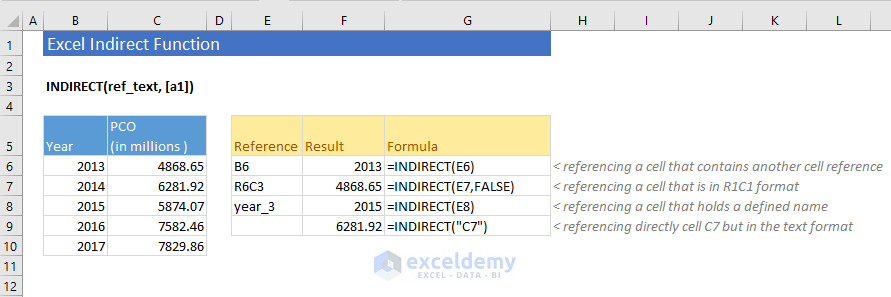Now let’s discuss how to use Excel to reference a cell in another worksheet based on cell value.

## Reference cells in another Excel worksheet based on cell value

I will show two examples here.

### Example 1: Select a single cell and refer a whole range of cells

I have two Excel worksheets with names BATBC and GP. You can have many. Both worksheets have similar kinds of data. Profit (PCO), EPS and Growth of two companies for the last 5 years.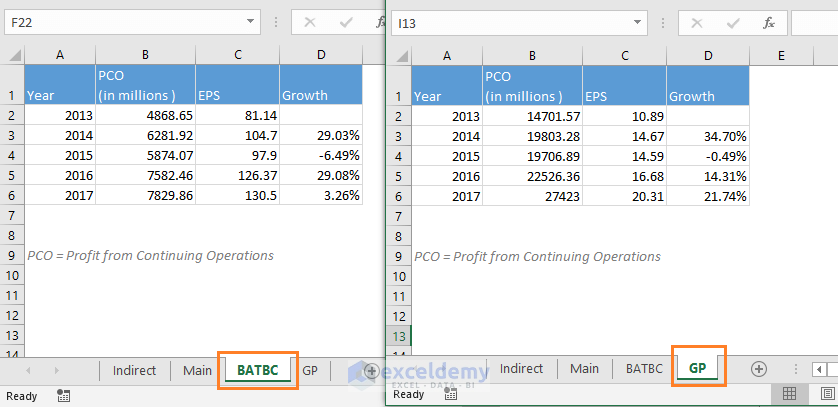What I want is: in the Main worksheet, I will input the company name from a drop-down and all those values (Year, PCO, EPS, and Growth) will be shown in the Main worksheet.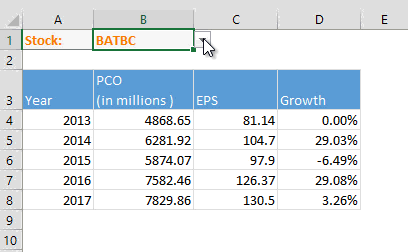This method can be helpful if you’re maintaining the same kind of data in hundreds of worksheets.

It is toilsome to search for every relevant worksheet and then view the data. For simplicity, I am using just two worksheets.

We shall use a formula like this:

=INDIRECT(‘worksheet_name’!column_reference&row_reference)

For column_reference, I have taken the help of this cell range: G5:K5. This range holds values from A to E. You can extend it as per your necessities.

For row_reference, I have taken the help of this cell range: F6:F14. This range holds values from 1 to 10. Extend it as per your necessities.

This is the real formula that I have used in the cell A4 of worksheet Main: `=INDIRECT("'"&\$B\$1&"'!"&G\$5&\$F6)`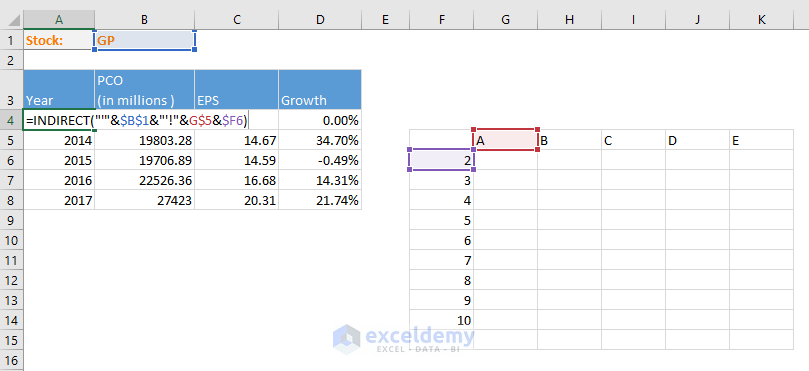Now apply this formula to other cells in the range (A4:D8).

This is the result you will get: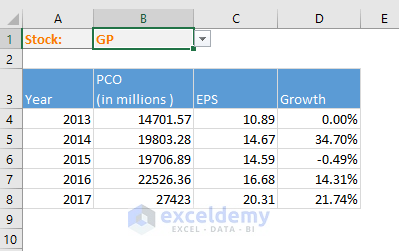How does this formula work?

• This part of the formula (`"'"&\$B\$1&"'!"`) returns the worksheet name `"'GP!'" `(for the above image)
• This part &G\$5 refers to cell reference G5 and returns text value `&"A"`
• And this part &\$F6 refers to cell reference F6 and returns numeric value &1
• So, this is the overall return from the 3 parts of the formula: `"'GP!'"&"A"&1 = "'GP!'A1"`

Note: When a text value and a numeric value is concatenated in Excel, the return is a text value.

• And here is the ultimate return of the formula: `INDIRECT("'GP!'A1")`
• When we apply the formula for other cells in the range, it works because we have used mixed cell references like G\$5 and \$F6. When the formula is applied to the cells on the right, only column references change for reference G\$5. When the formula is applied to the cells on the bottom, only row references change for reference \$F6.

Note: To see how the different parts of an Excel formula works, select that part and press the F9 key. You will see the value of that part of the formula.

### Example 2: Reference individual cell of another worksheet

In this example, I am pulling a row from another worksheet based on some cell values (references).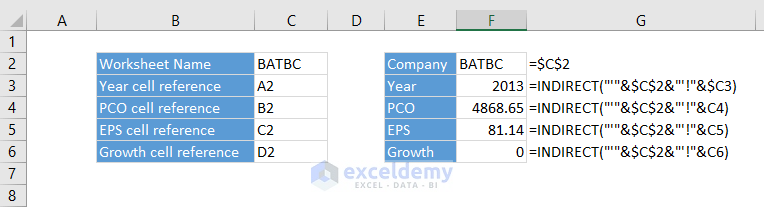I am showing this method as somebody might find it useful in his job.

If you want to another company and another year, you have to change 5 values. Check out this image now.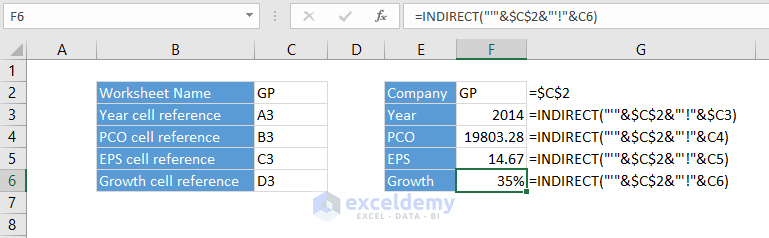I will not explain how this formula works as these formulas are the same as the above one.

## Conclusion

Hope this helps you out when you want to show data from a lot of worksheets in the main worksheet. Do you use any better method? Let me know in the comment box.

Hello!
Welcome to my Excel blog! It took me some time to be a fan of Excel. But now I am a die-hard fan of MS Excel. I learn new ways of doing things with Excel and share here. Not only how to guide on Excel, but you will get also topics on Finance, Statistics, Data Analysis, and BI. Stay tuned!

1. Replyjg Dec 15, 2018 at 3:04 AM

Great Article!!! I can put this to use right now 🙂 It would be even more powerful if I knew how to pull a table of data in from another worksheet or, even better, another workbook without the cell reference table being used as reference… For example I have similarly formatted data that I want to chart using the drop down as you have already done. To have this reference table, even hidden, will distract the people I will present the data to…

• ReplyDec 15, 2018 at 1:59 PM

Best regards
Kawser

2. Replyjohn ramsey Feb 10, 2019 at 6:25 PM

when a cell has a number (12345) that is ok but when the next cell has(\$123.45) how to get the decimal in
with out having to manual installing it.when there is ten cells part are numbers part are dollars.
i guess i will just put them ?

a b c d e
12 2 24 \$1.99 \$4.39
like this
jr

3. ReplyDave Oct 11, 2019 at 11:19 PM

=SUM(sheetname1!cell reference, sheetname2!cell reference,…)